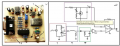# Designing circuit for measuring soil dielectric

#### arman74

Joined Jul 1, 2017
44
Hi.
Im trying to design a circuit for measuring soil dielectric.
I found such a circuit in some articles but i couldnt understand some parts.
I would be thankful if you help me.
The principle of the algorithm is using a pulse source of 50MHz and applying it to the soil probe and measuring the dielectric.

Here is the probe.Here is what i found.
In section 1 there is Rz and the soil prob.
In section 2 there is RLC circuit.
In section 3 there is rectifying diode.
My questions:
The oscillator is NTK so the supply is gnd and +5 volts. So the rectifying diode is always in forward bias?Or the RLC circuit cuts the dc and the diode rectifies the output of it?
How can a circuit with 0 and 5 volt input have a voltage under zero?
Should i power it with +-VCC to work?
How should i determine the component values?
What kind of oppamp should i use?
why is the signal buffered? Isnt the input impedance of the oppamp enough ?

Thanks a lot.

#### Delta Prime

Joined Nov 15, 2019
1,311
Hello thereRespectfully sir if I may.Volumetric contents of water in the soil scientifically is measured by the dielectric permittivity soil by means of a Coaxial Impedance Dielectric Reflectometry method which is far superior than the circuit you wish to use. and cost-effective.
if you have the capability I would seriously reconsider my optionsHello thereif you have the capability I would seriously reconsider my options